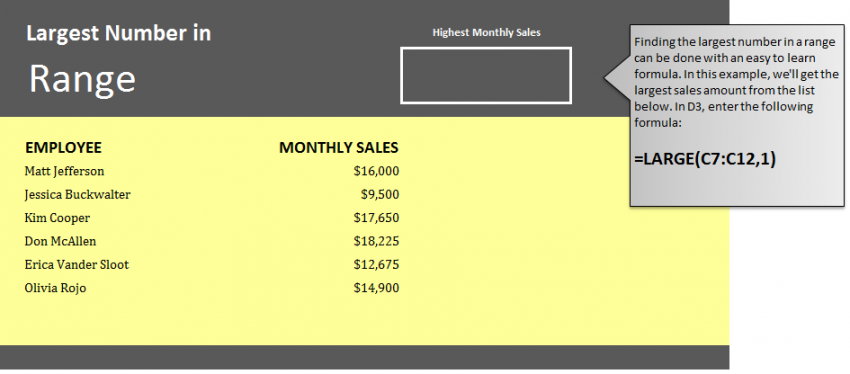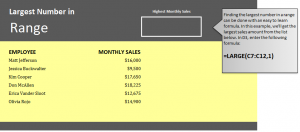# Get Biggest Number in Excel Range14
AprLearn how to find the biggest number in Excel worksheets. Excel has a formula that allows a user to select a group of cells and then enter the formula to automatically generate the correct answer of what the biggest number in that cell range is. This is a good tool to use when working with large amounts of data, especially spreadsheets that need you need to pull numbers from many different cell ranges. Learning how to correctly use this formula can help you when organizing employee data sheets, school grades, and many other spreadsheets.

Download the example page to learn how to find the biggest number in an Excel sheet.

Load the Excel sheet and you will be able to view two columns: One for employee names, and one for their monthly sales. Your goal in this example is to have Excel calculate what the highest monthly sales is and then generate the answer for you.

Select cell D3 and input the following:

=LARGE(C7:C12,1)

The result in D3 will show that Don McAllen’s monthly sales number of \$18,225.

The formula entered tells Excel to search column C between rows 7 through 12 for the highest number, and Excel searches these cells and returns the biggest number to cell D3.

Utilize this formula in your own Excel worksheets or practice more by changing the range of cells in the example.